A. Perturbations and Large-Scale Structure

During the inflation epoch, perturbations (small fluctuations) of two types are generated: scalar (density) perturbations, and tensor (metric) perturbations. The scalar perturbations come from quantum fluctuations of the inflaton field before and during its evolution. Tensor perturbations arise from quantum fluctuations in the space-time metric within the quasi-DeSitter spacetime. Fluctuations of this type are a natural consequence of a DeSitter spacetime.

In DeSitter space there exists an event horizon. Consider the distance light can travel in comoving coordinates, which is given by (6) and a(t) ~ exp(Ht).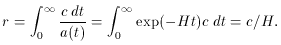(79)

The presence of this event horizon suggests the presence of thermal fluctuations in the fields, similar to those present in a black hole. This can be understood by appealing to the uncertainty principle. The event horizon causes the ground state modes of any fields present to be restricted in spatial extent. The uncertainty principle then requires,pH/c, since the characteristic size is just c/H. This uncertainty in momentum gives rise to energy fluctuations and the corresponding Hawking temperature is given by ,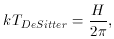(80)

where k is the Boltzman constant relating the energy to the temperature.

This result provides a motivation for the existence of fluctuations in the metric and scalar field. As these perturbations are created during inflation they are inflated outside of the causal horizon (particle horizon). As mentioned in the previous section, the causal horizon is nearly stationary during inflation. Once the perturbation has been inflated outside the horizon, its ends are no longer in causal contact. In this way, the perturbations become `frozen-in' as classical perturbations.

Ignoring the nonlinear, or super-horizon, effects of these perturbations may trouble the reader. However, as we shall see, ignoring these effects appears to be in agreement with the cosmic background data , , . As stated, one reason for choosing to ignore super-horizon evolution is the causal separation of the ends of the perturbation. However, this argument is far from rigorous and the study of nonlinear perturbations takes much care. Perturbation evolution relies on the extrinsic (super-horizon) properties of the spacetime manifold and is sensitive to the gauge of general relativity. The perturbation evolution can usually be ignored in regions where the pressure becomes negligible, which happens to be on the order of the horizon . This generally motivates one to ignore the super-horizon evolution, however for a complete treatment, see , , [5, Chapter 8 and 9].

After inflation the expansion continues at sub-luminal speeds and these perturbations enter back inside the causal horizon. Thus, the most important perturbations for creating structure come from the ones that were exited near the end of the inflationary period (i.e., approximately the last 60 e-foldings). After the reheating process occurs, the inhomogeneities passing back inside the horizon cause fluctuations that seed the large-scale structure.

Pure exponential inflation, which corresponds to a DeSitter spacetime, has an interesting property. The spacetime is invariant under time translation. That is to say, there is no natural origin of time under true exponential expansion [22, Chapter 11]. The only fundamental size in the theory is that of the Hubble horizon (c/H). Thus, one expects that the amplitude of a `standing wave' perturbation will be related to the horizon size, c/H, which is not changing. Therefore, we see why inflation predicts a scale-invariant spectrum for the perturbations.

This analysis can be illustrated through musical analogy. The fundamental mode of the perturbations are determined by the Hubble distance (c/H), much like the fundamental mode of a flute is determined by its length. Because the Hubble length (horizon) is nearly stationary during inflation, this means inflation predicts a scale-invariant, or Harrison-Zeldovich spectrum. Furthering this analogy, the `overtones` of the universe correspond to the inflaton potential that determines its behavior, much like overtones can be used to distinguish one instrument from another. However, as we have seen that inflation need not be exponential. The small deviations from DeSitter spacetime result in small deviations from a scale-invariant spectrum. These deviations can be used to successfully predict the correct potential for the inflaton.

The generated perturbations can be characterized by a power spectrum,H2(k). The H indicates that the perturbation amplitude is taken to correspond to its value when it crossed the causal horizon. Quantitatively, this corresponds to k = aH, where k is the wave number of the perturbation. Quantum field theory can be used to calculate an expression forH similar to Equation (80) above [22, Chapter 8].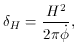(81)

where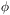is the inflaton. This formula manifests the connection between the inflaton potential and the perturbations generated during inflation.

One is generally interested in the scale dependence of the spectral index of these perturbations, since this dependence changes for different inflation models .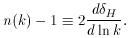(82)

For an absolute scale-invariant spectrum, it follows thatis independent of k and the above relation gives n = 1 as one would expect. For a spectrum that is nearly scale-invariant the amount n differs from one is referred to as the tilt of the spectrum. Although it is not at all obvious, (81) and (82) can be used along with the slow roll parameters (64), (63) to express the tilt as,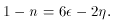(83)

The details of the calculation need not concern us here, for a derivation of this result see  or . This relation is only presented to demonstrate that the tilt, which is a discriminating factor between models, can be written in terms of the SRA parametersand. Therefore, if an experimental consequence of the tilt is observable, one can find the appropriate values for the SRA and reconstruct the inflaton potential .